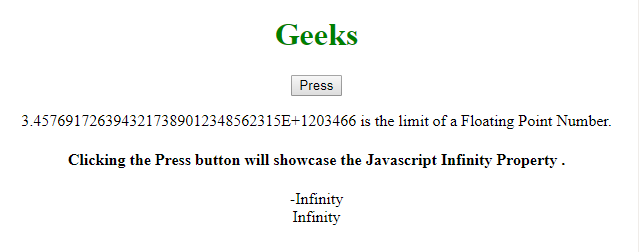Related Articles
JavaScript Infinity Property
• Last Updated : 06 Jul, 2020

Below is the example of the Infinity Property.

• Example:

 ` `

• Output:
```-Infinity
Infinity```

The Infinity Property used to display Infinity when the floating point number’s upper limit is exceeded and -Infinity when the floating point number’s lower limit is exceeded. Here, Infinity denotes Positive Infinity and -Infinity represents Negative Infinity.

Syntax:

`floating point no.`

Return Value: It returns Infinity to represent Positive Infinity and -Infinity to represent Negative Infinity.

More example code for the above property are as follows:
Program 1:

 ` ` `<``html``> ` `<``head``> ` `    ``<``style``> ` `        ``h1 { ` `            ``color: green; ` `        ``} ` `    `` ` ` ` ` `  `<``body``> ` `    ``<``center``> ` `        ``<``h1``> ` `          ``Geeks ` `      `` ` `        ``<``button` `onclick``=``"test()"``> ` `          ``Press ` `      `` ` `        ``<``p``> ` `          ``3.4576917263943217389012348562315E+1203466 is ` `          ``the limit of a Floating Point Number. ` ` `  `        ``<``h4``> ` `          ``Clicking the Press button will  ` `          ``showcase the Javascript Infinity Property. ` `      `` ` ` `  `        ``<``p` `id``=``"gfg"``> ` ` `  `        ``<``script``> ` `            ``function test() { ` `                ``document.getElementById( ` `                  ``"gfg").innerHTML = ` `                  ``-3.4576917263943217389012348562315E+1203466 + ` `                  ``"<``br``>" ` `                  ``+ 3.4576917263943217389012348562315E+1203466; ` `            ``} ` `        `` ` `    `` ` ` ` ` `  ` `

Output:Supported Browsers:

•